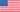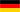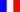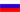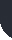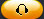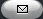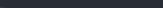Task Management Software for Team Collaboration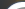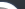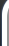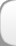HomeDownloadSupportPurchase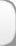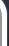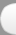Installation GuideStartUp GuideOnline Help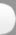# Phase Cost Growth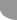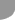Home  » Products  » VIP Task Manager Pro  » Tutorials  » Customization Guide  » Phase Cost Growth

Phase Cost Growth

Phase Cost Growth is a key performance indicator used in project management to define how actual cost per particular project phase changes over time comparing with baseline phase cost. This proportion expressed as percentage can be presented by the formula below:

Phase Cost Growth = (Actual Phase Cost - Baseline Phase Cost) / Baseline Phase Cost

Actual Phase Cost [Total Cost and Cost Factor for All Project Phases] is the total amount spent per certain project phase.

If project cost doesn’t involve Direct Material the formula to calculate Actual Phase Cost is below:

Actual Phase Cost = (Actual Phase Work * Standard Rate) + (Actual Phase Overtime Work * Overtime Rate) + Resource per Use Costs + Task Fixed Cost (actual per phase)

In contracting, Actual Cost for Materials and other expenses should be added and the formula is different:

Actual Phase Cost = (Actual Phase Work * Standard Rate) + (Actual Phase Overtime Work * Overtime Rate) + Resource per Use Costs + Actual Cost of Materials (per phase) + Other expenses (per phase)

Baseline Phase Cost is originally estimated cost per each project phase.
Baseline Phase Cost is calculated using the same values as for Actual Phase Cost:

For projects that do not involve Direct Material the following formula is used:

Baseline Phase Cost = (Baseline Work * Standard Rate) + (Baseline Overtime Work * Overtime Rate) + Resource per Use Costs + Task Fixed Cost (baseline per phase)

For construction projects the formula may be like this:

Baseline Phase Cost = (Baseline Work * Standard Rate) + (Baseline Overtime Work * Overtime Rate) + Resource per Use Costs + Baseline Cost of Materials + Other Expenses

Let’s consider an example when Baseline Phase Cost is \$6 000 USD and Actual Phase Cost is \$5 000 USD. Phase Cost Growth is calculated as follows:

\$5 000 USD - \$6 000 USD / \$6 000 USD = - 16.67 %

The value is negative. It means that the phase in question is on track and its budget is 16.67 % less than it was originally estimated.

The positive value would say that the project phase was beyond planned budget.

It is significant that, in contracting, for construction projects, for example, Phase Cost is calculated in the same way as Total Project Cost. Phase Cost for Owners is calculated based on the budget authorized before launching the project phase. Phase Cost for Contractors is the budget amount (baseline or actual) when contract is awarded.

Below are the instructions on how it is possible to calculate Phase Cost Growth using VIP Task Manager Professional:

• Add the custom field Baseline Phase Cost with Code “cf_baseline_phase_cost” and Type “Currency”. You may use one of the abovementioned formulas depending on the nature of your project. All values must be estimated amounts.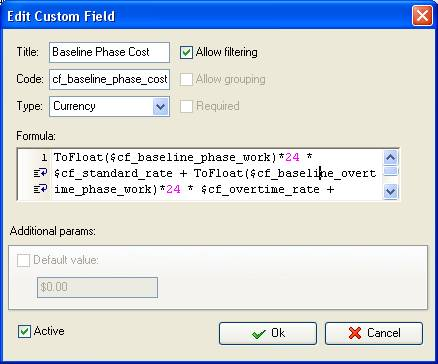• Add the custom field Actual Phase Cost with code “cf_actual_phase_cost”, Type “Currency” and similar formula with actual values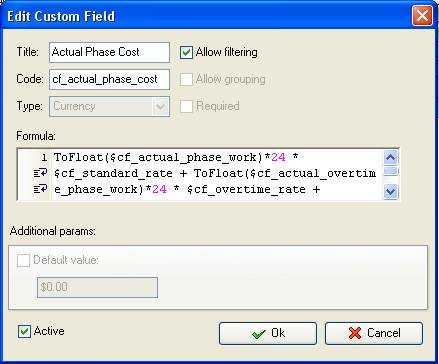• Add the custom field “Phase Cost Growth” with the formula:

(\$cf_actual_cost - \$cf_baseline_cost)/ \$cf_baseline_cost * 100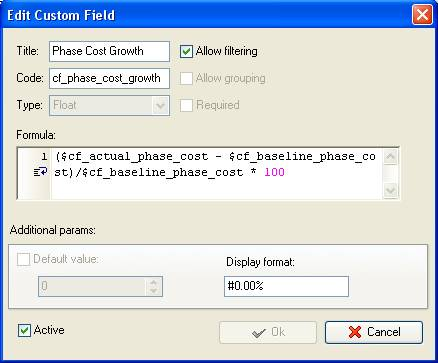• On Task Tree right click on the root task group and select “Custom fields options”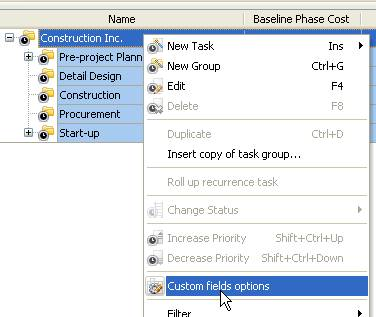• Set the following custom fields settings for the custom field “Baseline Phase Cost” and “Actual Phase Cost”

- In “Settings for” select “Subgroups&tasks”
- select “Enable”
- select “Sum” in “Aggregated (for task group)”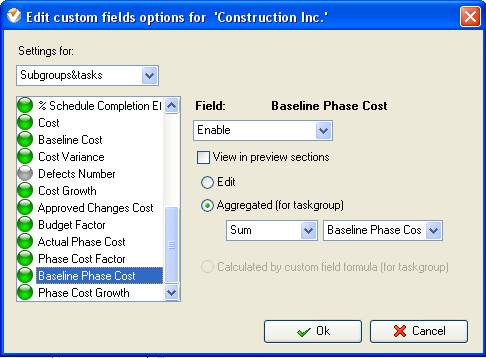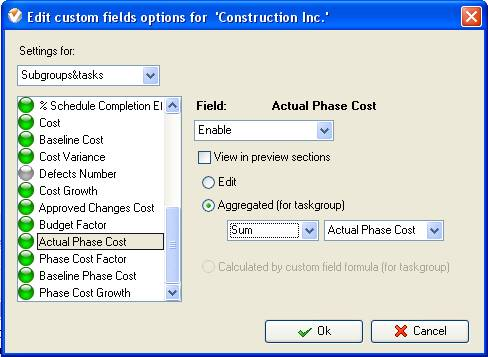• Right click on the root task group and set the following custom fields settings for the custom field “Phase Cost Growth”

- In “Settings for” select “Subgroups&tasks”
- select “Enable”
- select “Calculated by custom field formula (for taskgoup)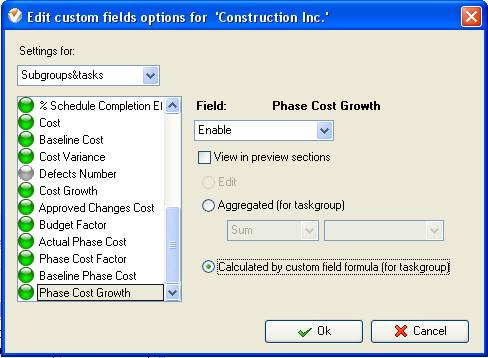Tip: to display the average cost growth for all project phases right click on the root task group and select “Average” for the custom field “Phase Cost Growth”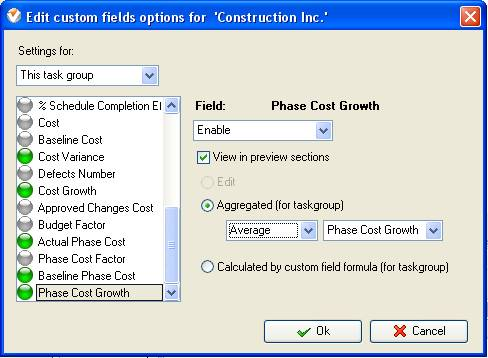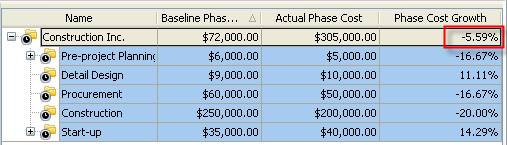Average Cost Growth for all Project Phases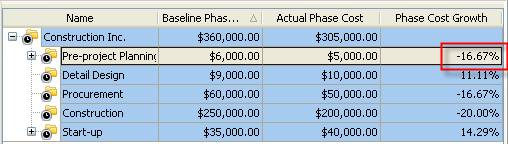Phase Cost Growth on Task Tree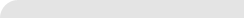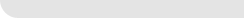» VIP Task Manager Pro VIP Task Manager Std Testimonials Turnkey Solution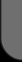ProductsSolutionsDownloadSupportPurchaseContactsForumVideoMy Account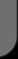Copyright © 2004 - 2023 Task Management Software